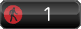## Thursday, January 03, 2008

### Logical-Mathematical Intelligence

Definition: Logical-Mathematical Intelligence is the capacity to reason, calculate, recognise patterns and handle logical thinking.

Usually found in: scientists, engineers, mathematicians, computer programmers, doctors, police investigators, researchers, accountants, economists, lawyers and animal trackers

Famous examples: Bertrand Russell, Marian Diamond, Bill Gates, Albert Einstein, Sir Isaac Newton

Logical-Mathematical Intelligence traits:

• likes to count
• likes to be organized
• is very precise
• good at problem-solving
• recognises patterns
• likes math games
• likes to experiment in a logical way
• orderly note-taking
• ability for abstract thinking
• likes computers

Exercises to strengthen logical-mathematical intelligence:

• Use deductive thinking like Sherlock Holmes
• Use computers for spreadsheets, graphs and calculations
• practice sequential step-by-step thinking!
• get organized and delegate a place for everything
• use prediction
• use reasoning
• play mathematical computation games
• do practical experiments
• analyse and interpret data and statistics

#### 0 komentar: